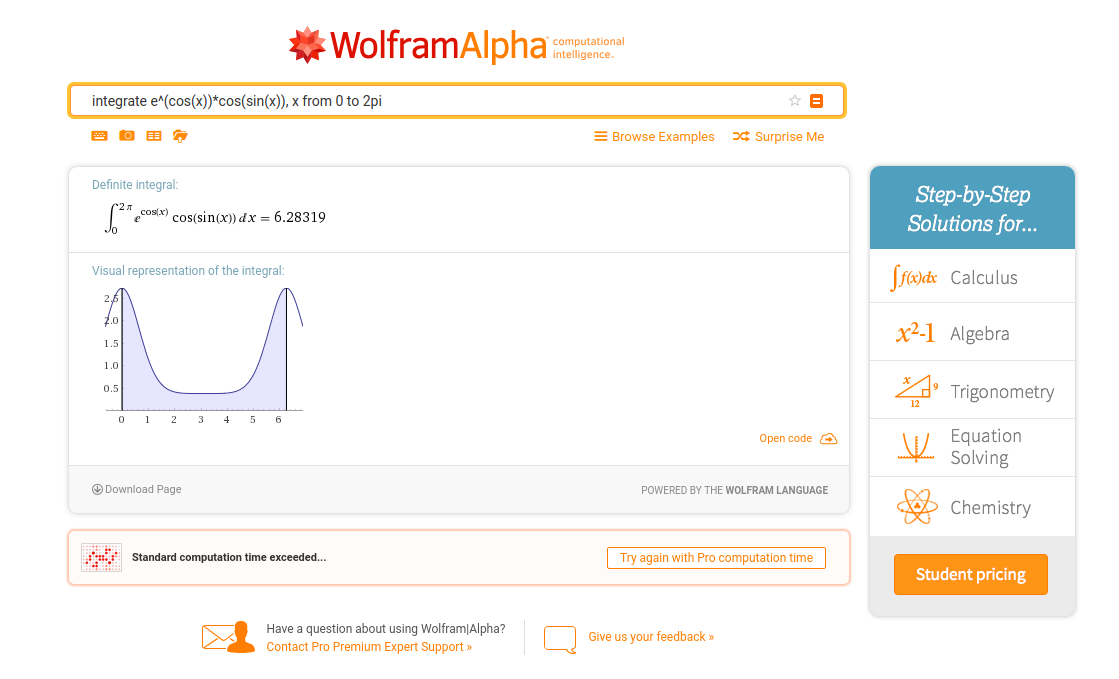# Having Fun with an Integral

2018-12-08

The main point is not quantity or speed—the main point is quality of thought.

–- Jane Gilman

$I = \int_{0}^{2\pi} e^{\cos \theta} \cos(\sin \theta)\; d\theta$

is an integral that WolframAlpha cannot YET compute analytically as of today (December 8, 2018). Here is the link.I think that there are two ways to tackle this problem by exploiting the analyticity of the integrand $e^{\cos \theta} \cos(\sin \theta)$. We can calculate it in the real domain by Taylor series or in the complex domain by Cauchy's integral formula.

### Solution by Taylor Series

First, let us introduce the function

$f(r) = \int_{0}^{2\pi} e^{r \cos \theta} \cos(r \sin \theta)\; d\theta,$

so we have the equation

$I = f(1)$

. By Taylor expansion, we have

$f(r) = \sum_{n=0}^{\infty} \frac {f^{(n)}(0)}{n!} r^{n}.$

It is easy to show that all the coefficients of this Taylor expansion vanish except for the first one. Namely, $f(0) = \int_{0}^{2\pi} e^{0 \cos \theta} \cos(0 \sin \theta)\; d\theta = 2\pi$. The $n$-th derivatives of $f$ at $0$ must be in the form of

$f^{(n)}(0) = \int_0^{2\pi} \sum_{k{\text{ even }}\wedge\; 0\le k\le n}(-1)^{\frac {k}{2}}{n \choose k}\cos ^{n-k}\theta \sin ^{k}\theta \; d\theta = \int_{0}^{2\pi} \cos(n \theta) \; d\theta = 0.$

Hence, we have $f(r) = 2\pi = I$.

### Solution by Cauchy's Integral Formula

First, let us introduce the same function

$f(r) = \int_{0}^{2\pi} e^{r \cos \theta} \cos(r \sin \theta)\; d\theta.$

The integrand can be converted to a complex exponential,

$e^{r \cos \theta} \cos(r \sin \theta) = \Re\{e^{r \cos \theta} \cos(r \sin \theta) + i \sin(r \sin \theta)\} = \Re\{e^{r\cos \theta + ir\sin \theta}\} = \Re\{e^{re^{i\theta}}\}.$

Cauchy's Integral formula at $0$ is

$g(0)=\frac{1}{2\pi i} \oint_\gamma \frac{g(z)}{z} \,dz.$

If we take $g(z) = e^z$, and $\gamma: re^{i\theta}$, we have

$g(0)=\frac{1}{2\pi i} \int_{0}^{2\pi} \frac{g(re^{i\theta}) ire^{i\theta}}{re^{i\theta}} \,d\theta = \frac{1}{2\pi} \int_{0}^{2\pi} g(re^{i\theta}) \,d\theta.$

Note that we have

$\Re\{g(re^{i\theta})\} = \Re\{e^{re^{i\theta}}\} = e^{r \cos \theta} \cos(r \sin \theta).$

Hence,

\begin{aligned} g(0) &= e^0 = 1 \\ g(0) &= \frac{1}{2\pi} f(r) \\ f(r) &= 2\pi \\ I &= f(1) = 2\pi. \end{aligned}